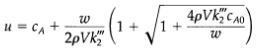### Create an Account

Home / Questions / Irreversible second order reaction in an agitated tank consider a system similar to that d...

# Irreversible second order reaction in an agitated tank consider a system similar to that discussed in Problem 23B 4 except that the solute disappears according to a second order reaction

Irreversible second-order reaction in an agitated tank consider a system similar to that discussed in Problem 23B.4, except that the solute disappears according to a second-order reaction; that is, RA,tot = – k'''2 Vc2A. Develop an expression for c A as a function of time by the following method:
(a) Use a macroscopic mass balance for the tank to obtain a differential equation describing the evolution of CA with time.
(b) Rewrite the differential equation and the accompanying initial condition in terms of the variable the nonlinear differential equation obtained in this way is a Bernoulli differential equation.
(c) Now put v = 1/u and perform the integration. Then rewrite the result in terms of the original variableCA.View less »

Jul 25 2020 View more View LessSubscribe To Get Solution